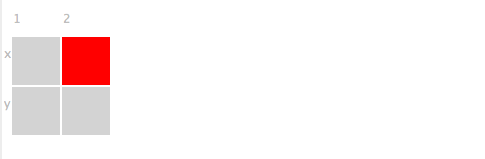### 4Using the Medic Debugger

Debugging with the Medic debugger involves three kinds of programs: source programs, Medic programs (by convention ending with “-medic.rkt”), and a program-starting script. Medic programs represent debugging instructions about the source programs and a program-starting script runs the Medic programs and starts debugging the source programs. After the evaluation of the program-starting script, a debugging graphical interface is presented, which consists of four panes: a Log pane, Graph pane, Aggregate pane and Timeline pane.

#### 4.1Tracing Log

Like the traditional print-like expressions, the tracing log produces linear and textual debugging information to identify problems in program execution. However, log is more advanced than the traditional print-like expression in three ways:
• Showing the context,

• Showing the behavior,

• Showing the layer of interest.

The content of the log entry produced by (log datum) varies with the datum type. If there is any context information about the datum that is available to the debugger such as the name of datum, it is displayed along with the value of datum. However, with the (with-behavior f template) definition in the Medic program, the logging behavior of f function calls is switched to displaying the behavior of the function f.

Suppose the value of x is 3 and we call (log x). Instead of merely printing out the value of x, it prints out “x = 3”, which displays the extra context information of the value 3—it is the variable x that we are inspecting.

All traditional print-like expressions are concerned with displaying values of data, but under some debugging circumstances, showing the behavior of data is needed. Consider the following example:

 (define (f x y) (+ (sqr x) (sqr y)))

When we call (log (f 3 4)), it produces a tracing log “(f 3 4) = 25”, which reveals no information about what the f function does. To change the behavior of (log (f 3 4)), we can modify the Medic program by adding
 (with-behavior f @{f: @x squared plus @y squared is @ret})
Then the call of (log (f 3 4)) generates “f: 3 squared plus 4 squared is 25”. Programmers have control over writing functions descriptions to obtain desired logging behaviors of function calls. The with-behavior forms allows programmers to change all logging behaviors of the same function call at different places just by changing the description at one place.

In general, traces become harder to understand with an increase of size and create a need for programmers to be able to focus on only the interesting parts of the trace. The layer Viewer feature of log offers a way to focus on relevant traces while preserving the execution order of traces.

The following source program traverses a tree to find the path to a desired node.
 #lang racket (define (find-path t name) (cond [(string? t) (if (equal? t name) '() #f)] [else (let ([left-p (find-path (cadr t) name)]) (if left-p (cons (car t) left-p) (let ([right-p (find-path (caddr t) name)]) (if right-p (cons (car t) right-p) #f))))])) (find-path '("a" ("b" "1" "2") ("c" "3" "4")) "3")
Suppose we want to insert some log expressions to see how the tree is traversed.
 #lang medic (layer left-path (in #:module "find-path.rkt" [at (if left-p _ _) [on-entry (log "left branch: ~a, ~a" (cadr t) left-p)]])) (layer right-path (in #:module "find-path.rkt" [at (if right-p _ _) [on-entry (log "right branch: ~a, ~a" (caddr t) right-p)]]))
We start a debugging session, and a trace browser is opened after the evaluation of Medic programs and augmented source programs.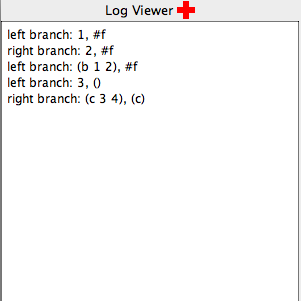What if we just want to see the path of left branches? By clicking on the “Log Viewer” button, a Layer Viewer window pops up, displaying check boxes of existing layer names.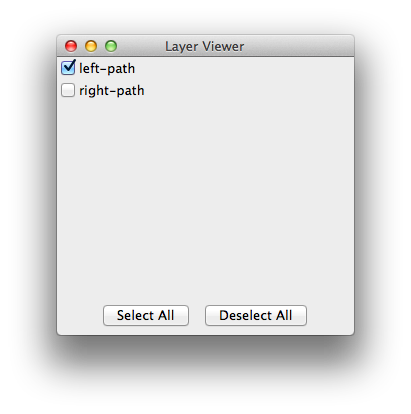Select the left-path check box, the Log Viewer is updated immediately, highlighting the traces which belong to the layer left-path.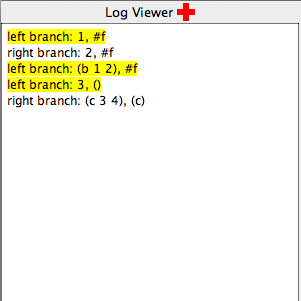#### 4.2Tracing Graph

A tracing graph presents a new means of tracing, allowing programmers to visually see the spatial relationship between trace elements. Text-based and linear traces can print out primitive values and preserve the execution order of programs but are limited for values that are reference types or compound data structures, and may exhibit connective relationship. The tracing graph eases the burden of programmers visualizing the spatial relationship mentally or drawing the graph manually on the paper by adding a lot of text-based tracing functions to print out the relationship, which is fundamentally textual and not visual. To avoid any overlap of graph nodes and achieve an aesthetically pleasing visual effect, the tracing graph uses force-directed algorithms for layout.

Here is one example illustrating the effectiveness of tracing graphs to find a bug in programs that is hard to uncover in text-based traces.

Suppose we have a correct implementation of a doubly linked list with support for common accessing, inserting, and removing operations. We comment out line 96 to create a bug.

Now we want to verify the correctness of the implementation. Traditionally, we would write the following Medic program to debug the source implementation.
 #lang medic (layer layer1 (in #:module "doubly-linked-list.rkt" [on-exit (define dlist (new doubly-linked-list%)) ; add ten elements (for ([i (reverse (build-list 10 values))]) (send dlist add-at 0 i)) (for ([i (in-range (send dlist get-size))]) (log "i=~a, datum=~a" i (send dlist element-at i))) ; remove five successive elements starting from the fourth element (for ([i (in-range 5)]) (send dlist remove 3)) (for ([i (in-range (send dlist get-size))]) (log "after removal: i=~a, datum=~a" i (send dlist element-at i)))]))

We are presented with a trace browser window containing a Log pane: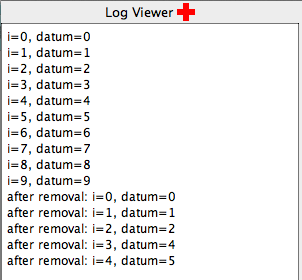It seems like the insertion operation with the list behaves correctly, but there is something wrong with the removal operation—the final list should be the sequence 0, 1, 2, 8, 9 instead of 0, 1, 2, 4, 5. The tracing logs give us little insight into the cause of the problem, and setting a breakpoint to step though the program and examine the previous and next references of each node requires a substantial amount of time. If we modify the Medic program by trying the tracing graph, we can see the problem instantly.
 #lang medic ;; disable this layer first (layer layer1 #:enable #f (in #:module "doubly-linked-list.rkt" [on-exit (define dlist (new doubly-linked-list%)) ; add ten elements (for ([i (reverse (build-list 10 values))]) (send dlist add-at 0 i)) (for ([i (in-range (send dlist get-size))]) (log "i=~a, datum=~a" i (send dlist element-at i))) ; remove five successive elements starting from the fourth element (for ([i (in-range 5)]) (send dlist remove 3)) (for ([i (in-range (send dlist get-size))]) (log "after removal: i=~a, datum=~a" i (send dlist element-at i)))])) ;; add a new layer using graph visualization (layer layer2 (in #:module "doubly-linked-list.rkt" [on-exit (define dlist (new doubly-linked-list%)) (for ([i (reverse (build-list 10 values))]) (send dlist add-at 0 i)) (for ([i (in-range 5)]) (send dlist remove 3)) (for/fold ([temp (get-field head dlist)]) ([i (in-range (sub1 (send dlist get-size)))]) (define next (get-field next temp)) ; draw an edge from the current node to its next referencing node with the red arrow color (edge temp next "" "Red" (get-field datum temp) (get-field datum next)) next) (for/fold ([temp (get-field next (get-field head dlist))]) ([i (in-range (sub1 (send dlist get-size)))]) (define prev (get-field previous temp)) ; draw an edge from the current node to its previous referencing node with the default gray arrow color (edge temp prev "" #f (get-field datum temp) (get-field datum prev)) (get-field next temp))]))
We restart the debugging session and the trace browser is opened where the edges and nodes are visualized in the Graph pane. From the graph, we can visually notice that the doubly linked list is broken: a correct list should have the property that every edge between nodes is bi-directed. After the first remove operation, the previous reference of node 4 is still pointing to the old node 3. Through the graph visualization, we can narrow the problem to an incorrect reference update using the remove method, and the information leads us to go back to the relevant code in the remove implementation and find the bug.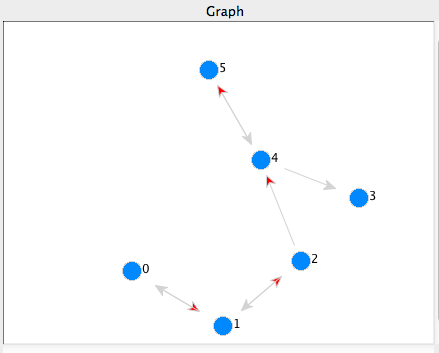#### 4.3Aggregate View

The aggregate view tackles the problem of grouping multiple trace elements that may be spatially separated in the source program or are relevant to each other, for example in the same control flow of the program or belonging to the same category of features. Also it supports scrubbing and data comparisons diff.

To illustrate, the source program and Medic program are as follows.
 #lang racket (define (fact x a) (if (zero? x) a (fact (sub1 x) (* x a)))) (fact 3 1)
 #lang medic (layer layer1 (in #:module "fact-iter.rkt" [(fact) [on-entry (aggregate x a)]]))

The Aggregate pane of the trace browser displays the following aggregate view of data, where the values of x and a are grouped together in each column.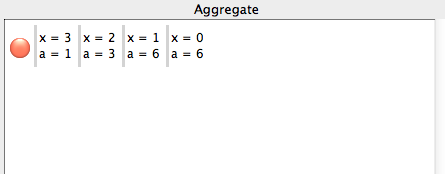If traces grow overwhelming, we can click on the light-red circle button to the left of the entries and open a scrub view window. The scrub view allows us to focus on values at one step by scrubbing through the traces recorded in the temporal dimension.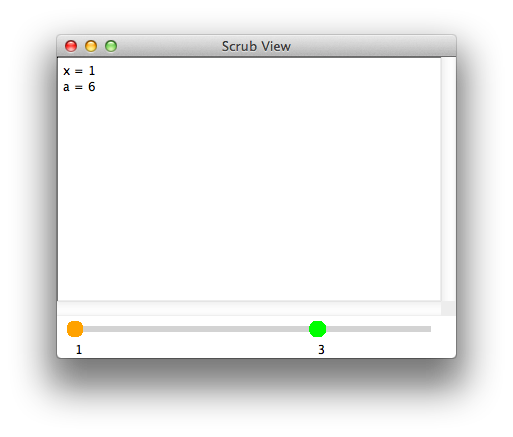For data comparisons, we can right-click on either of the two slider handles—turning red when right-clicked—to mark the step of data to which we want to compare the current values. Differences between two steps of data are highlighted in pink.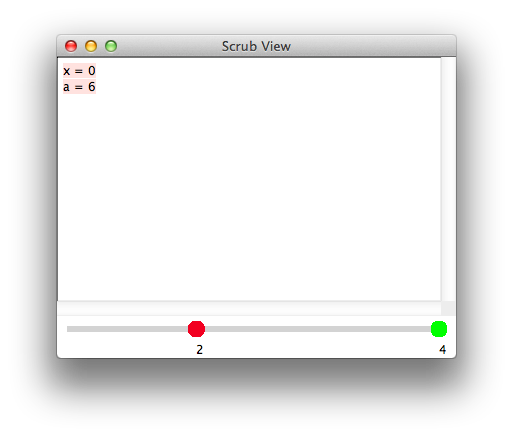#### 4.4Timeline View

The timeline view focuses on showing the panorama of individual trace elements. It allows programmers to get an overview of the pattern of changes of values over time at a glance and examine values of interest. The vertical axis of the Timeline pane records each trace element and the horizontal axis represents values of each trace element over time. There is a timeline slider on the top of the Timeline pane. The timeline slider can step through the timeline showing multiple values at the same horizontal coordinates at the same time. Clicking on any timeline square unit pops up a tooltip window showing current individual value.

• (timeline v)

If the data types of v over time are all numbers, a line plot is rendered on the timeline. For boolean values, the timeline is composed of colored square units, red denoting false values and blue denoting true values. For other mixed data types, the literal value is displayed.

One example:
 #lang racket (define (count-length v count) (if (null? v) count (count-length (cdr v) (+ count 1)))) (count-length (cons 8 (cons 9 '())) 0)
 #lang medic (layer layer1 (in #:module "count.rkt" [(count-length) [on-entry (timeline count) (timeline v) (timeline (null? v))]]))
The timeline: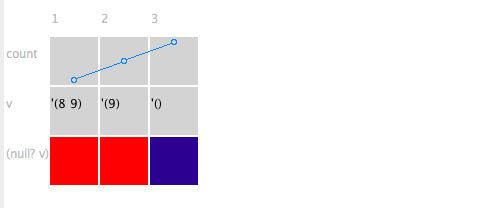The timeline slider: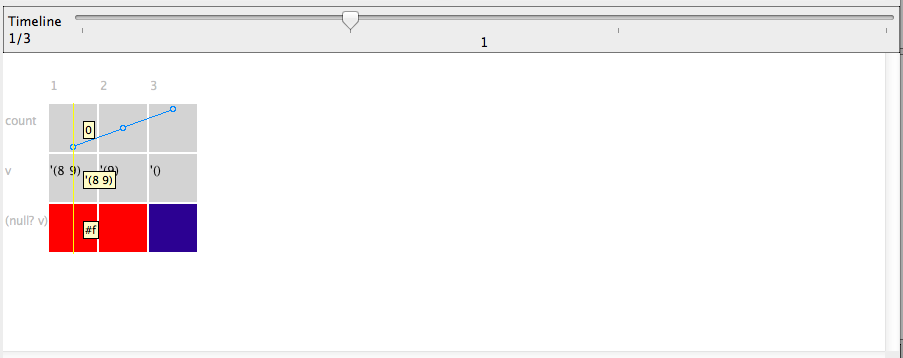• (assert pred)

Asserts pred to be true. If it fails, the square unit corresponding to the failed value is highlighted in red. Other square units remain the default gray background color.

One example:
 #lang racket (define (fact x) (if (zero? x) 1 (* x (fact (- x 1))))) (fact 3)
 #lang medic (layer layer1 (in #:module "fact.rkt" [(fact) [on-entry (assert (> x 0))]]))
The timeline: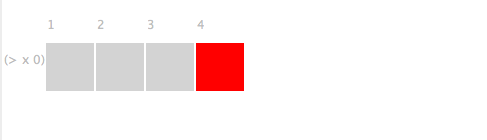• (same? v)

Traditional debuggers are usually only concerned with primitive data values. We define the state of a compund data element as a permutation of possible states of the primitive data members. If programmers want to know whether the state of a compound data changes or not, they need to memorize the previous histories of primitive data members, examine all the current primitive data members at a micro level and make comparisons, which requires a significant amount of debugging effort. The Medic debugger saves the debugging work that programmers have to do mentally and manually by treating the compound data of interest as a unit, the same as the primitive data in the source program, and monitoring value changes at a macro level.

When (same? v) is called for the first time, it always returns true. Aside from the first call, if the current state of v is different from the previous state, the call returns false; otherwise, the value is true. Like (assert pred), only false values are highlighted in red in the timeline.

Here is one debugging example.

 #lang racket (define x (vector 1 2 3 4 5)) (define y (list 1 -2 3 4 -5)) (define (convert-to-vector v) (cond [(vector? v) v] [(list? v) (apply vector v)])) (define (make-new-vector v) (vector-set! (convert-to-vector v) 0 #f)) (make-new-vector x) (make-new-vector y)
The source program intends to create a new vector based on the original array structures without modifying them. In order to verify that the old array structures remain unchanged, a Medic program with same? is added.
 #lang medic (layer layer1 (def check-changed #:src (same? x) (same? y)) (in #:module "same.rkt" [(at (define y (list 1 -2 3 4 -5))) [on-exit (ref check-changed)]] [on-exit (ref check-changed)]))
In the Timeline pane, we can clearly see that the source program is erroneous, because the value of x is changed.